# Rubik's Magic: Create the Cube

Create the Cube puzzle

# Create the Cube puzzle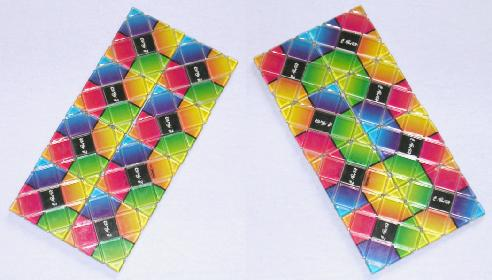This puzzle is mechanically exactly the same as the normal 2×4 version of Rubik's Magic, the only difference is in the design on the two sides. Each tile is split into four smaller squares which are coloured (blue, yellow, red, and green), and each tile uses all four colours on both sides. The aim of this puzzle is to turn it into a cube which is balanced on an edge on top of a stand made from two tiles. This must of course be done in such a way that the colours of adjacent tiles match (except for the underside of the 2 tiles it stands on).

If you have a normal Rubik's magic, you can convert it to the Create the Cube puzzle, either by sticking on some coloured labels (avoiding the grooves of course), or if you are patient you can undo the wire loops (do not cut them, just stretch them over the nearest corner), replace the paper squares and then refit the wires. See the section on making your own Magic for details.

## Solution to Create the Cube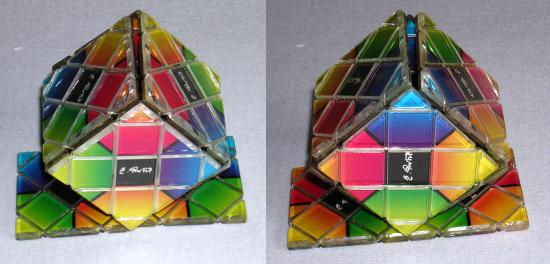If you examine the two sides of the puzzle, you will notice that on one side, the front, all the tiles have the same colour combination (yellow, green, red and blue going clockwise around the tiles edge). In the starting position the front tiles will be oriented the same way.
On the back side of the puzzle the colour combinations of the tiles mostly differ. This side will eventually become the outside of the cube when it is solved.

There are in fact two solutions to this puzzle. First the puzzle has to be brought to one of two correct rectangular positions, and then the rectangle has to be changed into the cube shape. Follow the following steps to get one of the correct rectangular positions starting from any other rectangular position.

1. Turn the puzzle over, so that the side with the differing colour combinations is facing you.
2. Find the two identical tiles that show the colour combination YGBR looking clockwise around the tiles' edge. The two tiles will be diametrically opposite each other.
3. The two tiles should be in the top right and bottom left corners (when holding the rectangle horizontally, i.e. it is 4 wide and 2 high). If not, then do the star shift transform to bring the two tiles to those positions.
4. There is another pair of identical tiles (YRBG). These should be adjacent to the bottom left corner. If not, then rotate the puzzle through 180 degrees.
5. If the colour in the bottom left corner is blue or yellow, then do the rectangle transform, and start again at step b.
6. Look at the point where the four tiles on the left meet. They should all show the same colour there (red or green). If not, then do the rectangle transform, the star shift transform, and then the rectangle transform again, and go back to step b.
7.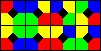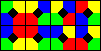You should now have one of the two correct patterns. You can swap between the two patterns by using the loop shift transform, shifting the top row one tile to the right. To turn this into a cube, follow the steps in the following section.

I find it interesting that from the starting position with all the red corners pointing towards the top left, applying the twist transform will bring it to one of the correct positions for making the cube. This shows that the front and back designs have exactly the same relation to each other as on the normal magic.

## Making the cube shape

One of the most elusive shapes for the Rubik's Magic is the cube. Six tiles form the cube shape and it stands on one edge on top of the other two tiles. There are actually three possible cube configurations, plus their mirror images. Only one of these configurations is a solution to the Create The Cube puzzle. I will show two methods for solving the Create The Cube puzzle, and also methods for reaching the other two cube configurations. The first method below will solve the Create the Cube puzzle provided you start from one of the two positions mentioned above.

1.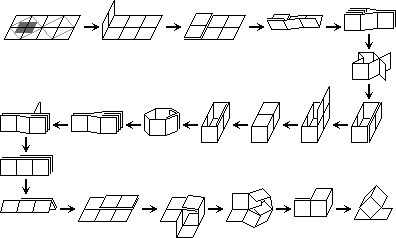Start from the 2×4 rectangle shape. If you are using a standard magic, hold it with the solved linked rings side on top. Fold two leftmost tiles up on top of the adjacent two.
2. Fold the 2×3 in half upwards, which places the two previously folded tiles inside, stacked together.
3. Open up the stacked tiles to form a 6-tiled loop, with two tiles stuck to the side of it, looking a bit like a fish shape. If this does not happen, and the stacked tiles can only fold up or downwards, then go back to the starting position, turn the puzzle over and try again.
4. Arrange the 6-tile loop into a 1×2 rectangular open box, with the extra tiles separated and folded against the long side of the box.
5. Fold the two extra tiles up, until they form a lid for the box.
6. Push this lid through into the inside of the box until it lies flat against the side. This is somewhat stressful for the wires, so be careful.
7. Stretch the box flat, which stacks together the lid tiles inside.
8. Fold one tile from the stacked end to the middle. On the Create the Cube puzzle you must fold the rear tile to the left, not the front tile. The two newly visible tile sides should have matching colours.
9. Fold open the puzzle into a 2×3 rectangle. The corner tile on the far right has an extra tile stacked on top of it, as does an adjacent tile.
10. Keeping the middle pair of stacked tiles together, open the other pair of stacked tiles to form a kind of square tube.
11. Open the remaining stacked tiles so that they hinge at one end of the tube, and the puzzle will strangely fold up into a cube shape with a handle of two stacked tiles.
12. Separate the two tiles in the handle to make a 2×1 stand for the cube, and set the puzzle down on them.

## Making the Cube, Solution 2: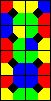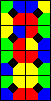There is another way to reach the same cube configuration. This method starts with one of two starting positions that differ from the previously mentioned ones. These have four adjacent tile corners which are either all blue or all yellow. These can be reached by much the same method as before, except that in step e, the rectangle transform should be done if the bottom left corner is NOT blue or yellow. In step f the four tile corners should again show the same colour, but it ought to be blue or yellow instead. Hold the puzzle flat with the blue or yellow spot nearest to you, and do the following moves.

1.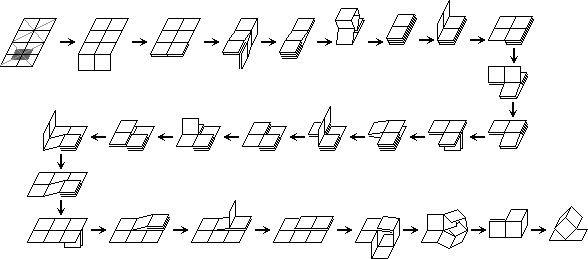Fold the two tiles nearest to you down underneath the adjacent two.
2. Fold the 4 tiles in the right half down underneath the left half.
3. Open up the tiles in a 6-tile loop and fold it up to make a four layer block. The two tiles that are not part of the loop remain pointing away from you at the bottom.
4. Fold the two tile top layer up to the left.
5. Fold the nearest top two tiles away from you on top of the two furthest tiles.
6. Fold the bottom two tiles at the far right corner down towards you underneath the nearest tiles.
7. Fold the two tiles on the right up to the left onto the left tile.
8. Fold the far left tile up towards you onto the front left tile.
9. Fold the left two tiles up to the left, making a 2×3 rectangle.
10. Fold the bottom two tiles at the front right down underneath the far right corner.
11. Fold the top tile at the far right leftwards on top of the adjacent tile. You now have a 2×3 rectangle with tiles stacked on top at the right and middle of the far side.
12. Keeping the middle pair of stacked tiles together, open the other pair of stacked tiles to form a kind of square tube.
13. Open the remaining stacked tiles so that they hinge at one end of the tube, and the puzzle will strangely fold up into a cube shape with a handle of two stacked tiles.
14. Separate the two tiles in the handle to make a 2×1 stand for the cube, and set the puzzle down on them.

## Making the Cube, other configurations:

The following method is much more stressful on the wires, but it is the most straightforward way to reach the other two possible cube configurations. The second of these configurations is very similar to the one above. If performed on the Create the Cube puzzle, it can make the cube shape with the matching colours on the inside of the cube!

1.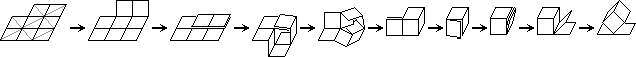From the rectangle shape, do the six-flip shape changing transform.
2. Continue the final movement of the transform above, bringing the flap of two tiles right round to the back of the other tiles. Then turn over the puzzle.
3. You now have a 2×3 rectangle. The middle tile on one side has an extra tile on top of it, as does an adjacent corner. Keeping the middle pair together, open the other pair to form a square tube.
4. Open the remaining stacked tiles so that they hinge at one end of the tube, and the puzzle will strangely fold up into a cube shape with a handle of two stacked tiles.
5. You can open up the handle to form a basket shape at this point.
6. Fold the basket handle back again so you get a cube with a handle of two stacked tiles, and fold the two tiles of the handle onto the side of the cube that is made from the third tile of the basket handle. This is where the wires begin to feel the strain, because the inner two tiles of the stack have to squeeze between those forming the sides of the cube.
7. Fold the two tiles on the inside of the stack through the inside of the cube onto the inside of another tile, to make another stack of three.
8. Fold the two tiles on the outside of the stack out to form a 1×2 stand, and place the puzzle down onto it.
9. To reach the other cube configuration, fold the platform tiles together. One of the two tiles together with one of the cube's sides can fold open to give an open box with three tiles around the rim. Now fold the other tile to close the box, and open out the remaining two tiles as a stand.